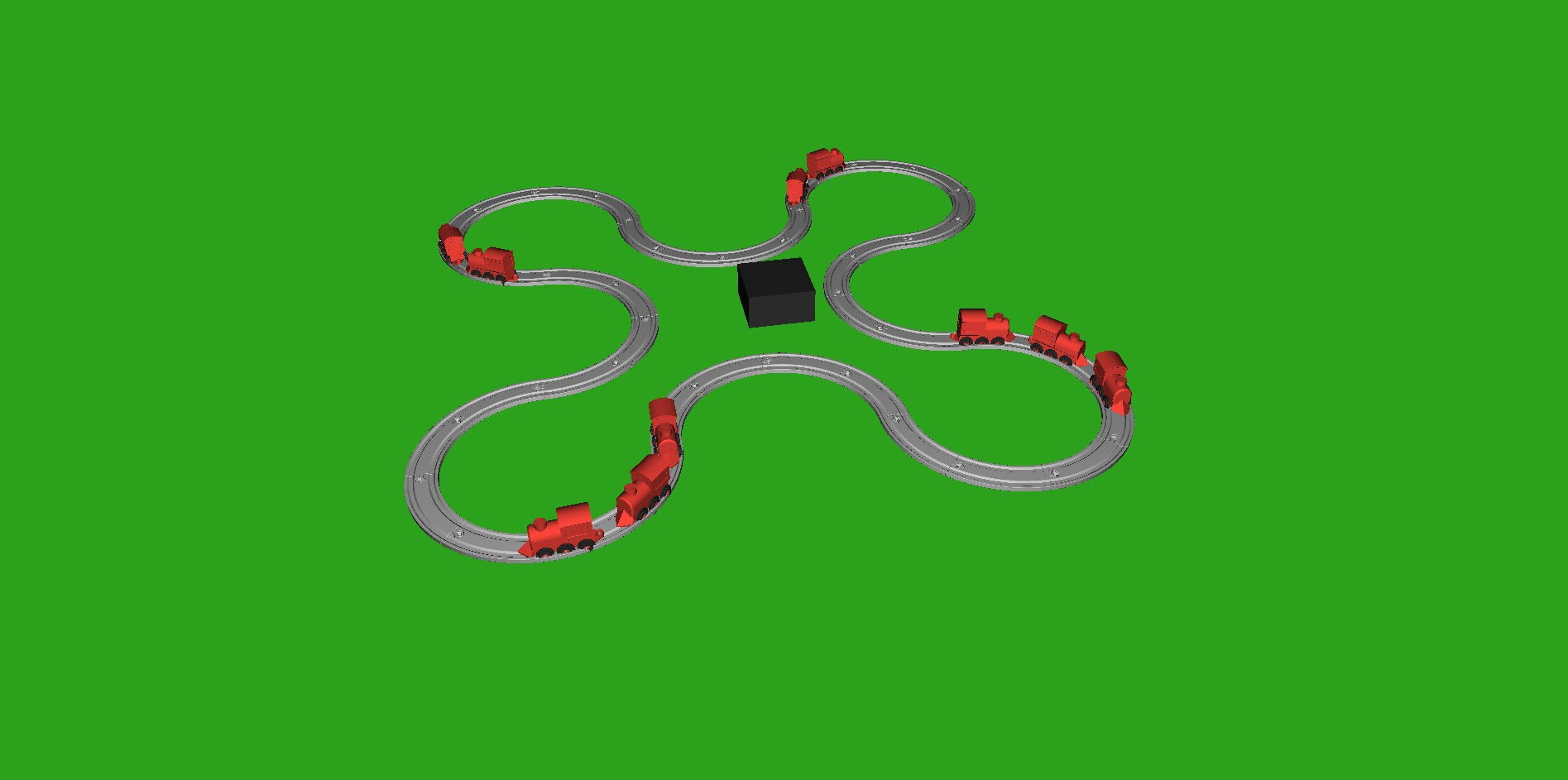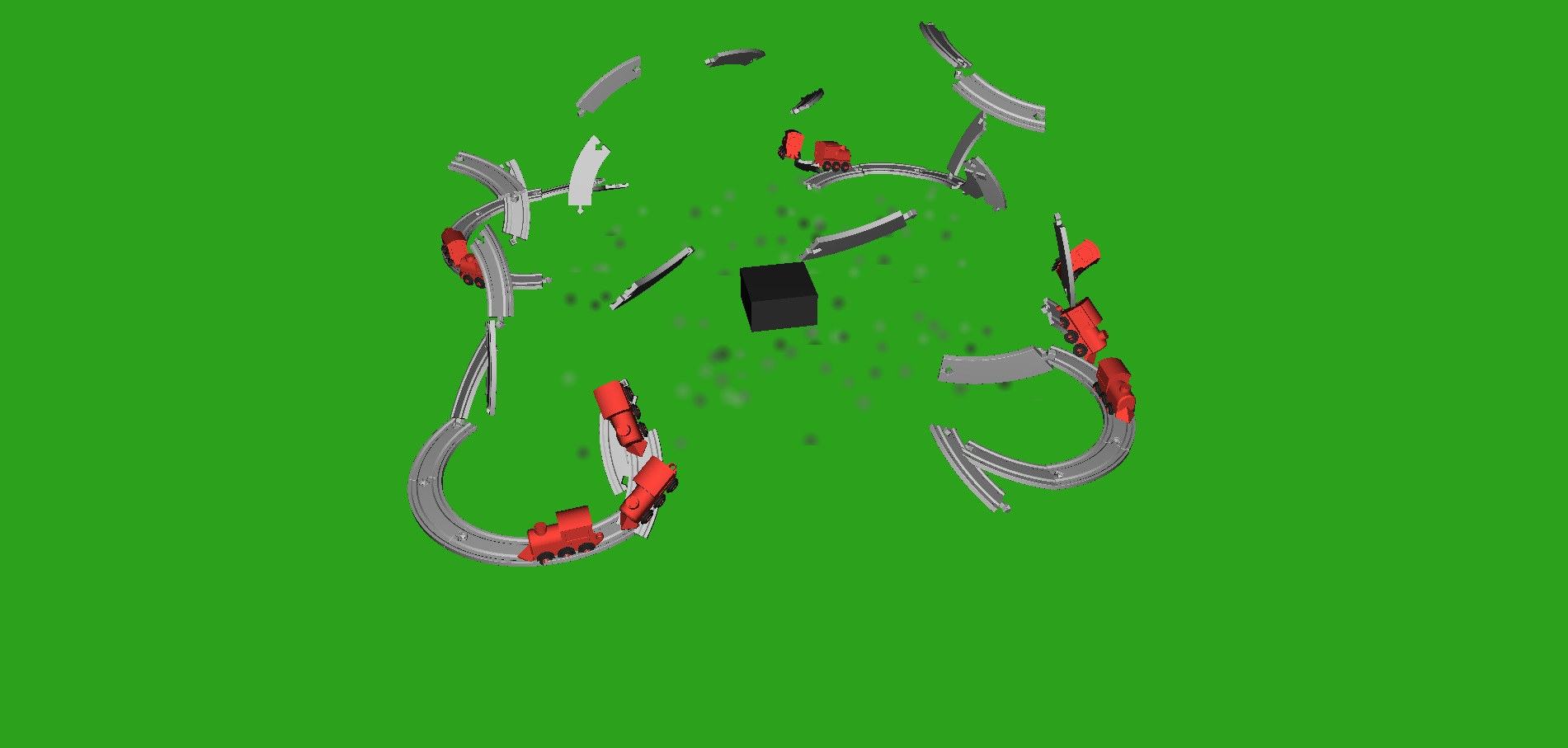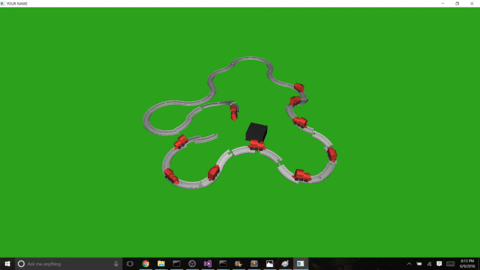# Overview

The Brio Train Explosion Simulator allows users to set up a brio scene and then ... blow it up. The simulation uses rigid body dynamics with floor collision, box-box collision, and friction.

# Features

The brio Train Explosion Simulator can be configured in the following ways:

• The user can set up the scene with any track from a configuration file.
• The user can set the number of trains.
• Trains will automatically be placed on the track.
• The user can choose explosion point in real time.
• The user can reset the scene.
• The user can create an explosion point multiple times in a scene.# Method

All of the explosion physics are done with rigid body dynamics with an added external explosion force and gravity. All objects can collide with each other. To do this, all objects are bound in a tight bounding box.

## Rigid Body Dynamics

### Collisions

All objects can collide with the floor and the ground. To do this, I create a contact matrix that is constructed on each time step. Every line of the contact matrix is one collision. The goal is to force the velocity of the object to point along the normal direction of the hit object.

For the ground, this is simply {0,1,0}, but for box to box collision, I use odeBoxBox.cpp which calculates the collision point between two blocks, as well as the collision normal.

Because collisions create an inequality constraint for the bodies, I use Mosek's Quad Prog function to solve for the new velocity of the bodies.

### Friction

Without friction simulation, all tracks and trains will collide and slide on the ground without appropriate loss of energy. I simulate friction by calculating the tangents to the normal of the collision and the relative velocity of the two colliding objects. This is then used to calculate a 6x6 portion of a stiffness matrix, "K". I use "K" to aid in the calculation of the next velocity of the object. Friction damping is used to control the power of the friction on the rigid bodies. I use a damping of 20, which is much lower than the examples used in class, but it looks the most natural with the current scenarios.

## Explosion Force

To get the tracks and trains flying, I create a small blast force with a radius of 5. Only objects within this radius are affected by the force. First, the direction of the force is calculated by subtracting the vector location of the body by the vector location of the explosive. In order to make objects fly up, I put the location of the blast force a bit below the ground.

After calculating the direction of the force, the impulse magnitude is calculated by multiplying the overall blast power by the squared inverse distance between the objects. This causes the blast to have less power for objects that are farther away. I also cheat a little bit by faking an angular velocity based on the force direction and distance.

This external force is added to the other external forces, such as gravity, when calculating the overall force of the body in each step.

# Resources

• Rigid Body Lecture Material
• Windows 10 for all models including train, and tracks
• Eigen
• Mosek